# Nonlinear Model Examples[Page 463]

In our previous profit analysis examples, the models followed the traditional mathematical (i.e., linear) programming formulation, with an objective function, decision variables , and constraints. However, many types of problems employ well-known nonlinear functions but are not typically thought of in the traditional math programming model framework. In this section we consider several additional examples that include familiar nonlinear functionsthe facility location model and the investment portfolio selection model.

#### Facility Location

In the facility location problem, the objective, in general, is to locate a centralized facility that serves several customers or other facilities to minimize distance or miles traveled between the facility and its customers. This problem uses as a measure of distance the formula for the straight-line distance between two points on a set of x, y coordinates, which is also the hypotenuse of a right triangle: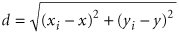where

( x, y ) = coordinates of proposed facility

( x i , y i ) = coordinates of customer or location facility, i

Consider, for example, the Clayton County Rescue Squad and Ambulance Service, which serves five rural towns, Abbeville, Benton, Clayton, Dunning, and Eden. The rescue squad wants to construct a centralized facility and garage to minimize its total annual travel mileage to the towns. The locations of the five towns in terms of their graphical x, y coordinates, measured in miles relative to the point x = 0, y = 0, and the expected number of annual trips the squad will have to make to each town are as follows :

Coordinates

Town

X

Y

Annual Trips

Abbeville

20

20

75

Benton

10

35

105

Clayton

25

9

135

Dunning

32

15

60

Eden

10

8

90

The objective of the problem is to determine a set of coordinates ( x, y ) for the rescue squad facility that minimizes the total miles traveled to the town, according to the following function: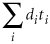where

d i = distance to town i

t i = annual trips to town i

[Page 464]

The Excel solution to this problem is shown in Exhibit 10.12. The coordinates ( x, y ) for the new rescue squad facility are in cells C14:C15 . The formulas for the distances between the rescue squad facility and each of the towns ( d i ) are in cells E6:E10 . For example, the formula for the distance, d A , between the rescue squad facility and Abbeville in cell E6 is =SQRT (( B6C14 )^ 2 +( C6C15 )^ 2 ). The formula for the total annual distance in cell D18 is =SUMPRODUCT ( E6:E10,D6:D10 ), which is also shown on the formula bar at the top of the spreadsheet. In the Solver window for this problem, D18 is the target cell that is minimized, and the changing cells (i.e., decision variables) are C14:C15 . There are no constraints.

##### Exhibit 10.12.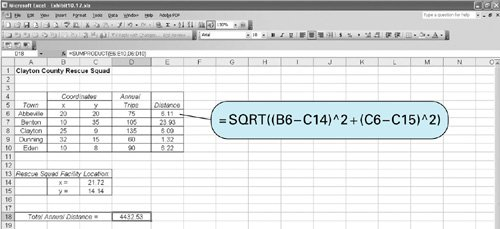The location of the rescue squad facility is shown graphically in Figure 10.8.

##### Figure 10.8. Rescue squad facility location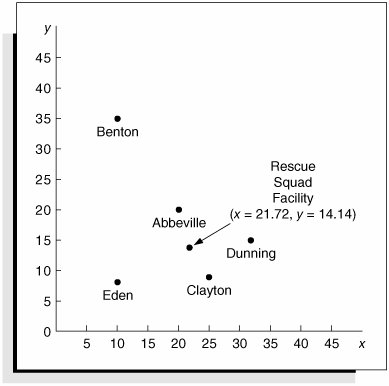[Page 465]

#### Investment Portfolio Selection

A classic example of nonlinear programming is the investment portfolio selection model developed by Harry Markowitz in 1959. This model is based on the assumption that most investors are concerned with two factorsreturn on investment and risk. Thus, the objective of the portfolio selection model is to minimize some measure of portfolio risk while achieving a minimum return on the total portfolio investment. Risk is reflected by the variability in the value of the investment, and in this model, variance in the return on investment is the measure of risk.

Covariance, which is a measure of correlation, is also used to reflect risk. Individual investment returns within a portfolio typically exhibit statistical dependence. Over time, the returns of any two stocks may exhibit positive or negative correlation; that is, two stocks of the same general type will go up or down together. To adjust for this possible correlation, investors often attempt to diversify their portfolios. To reflect the risk associated with not diversifying, the model includes covariance.

The minimization of portfolio risk, as measured by the portfolio variance, is the model objective. The variance, S , on the annual return from the portfolio, is determined by the following formula: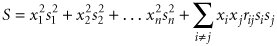where

x i , x j = the proportion of money invested in investments i and j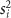= the variance for investment i

r ij = the correlation between returns on investments i and j

s i , s j = the standard deviations of returns for investments i and j

In the preceding formula for S , the first part is a measure of variance, and the second part is a measure of the covariance. In many cases, the parameters in this equation may be estimates or sample values (the sample variance, sample covariance, etc.).

The general formulation of the portfolio selection model is as follows. The investor desires to achieve a minimum expected annual return from the portfolio, which is formulated as a model constraint as follows:

r i x i + r 2 x 2 + . . . + r n x n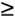r m

where

r i = expected annual return on investment i

x i = proportion (or fraction) of money invested in investment i

r m = minimum desired annual return from the portfolio

A second constraint specifies that all the money is invested:

x 1 + x 2 + . . . + x n = 1.0

The following example and Excel solution will demonstrate how this model is applied. Jessica Todd has identified four stocks she wants to include in her investment portfolio. She wants a total annual return of at least .11. From historical data, she has estimated the average returns and variances for the four investments as follows:

[Page 466]

Stock ( x i )

Annual Return ( r i )

Variance ( s i )

1. Altacam

.08

.009

2. Bestco

.09

.015

3. Com.com

.16

.040

4. Delphi

.12

.023

She has also estimated the covariances between the stocks, as follows:

Stock Combination ( i , j )

Covariances ( r ij )

A,B (1,2)

.4

A,C (1,3)

.3

A,D (1,4)

.6

B,C (2,3)

.2

B,D (2,4)

.7

C,D (3,4)

.4

## Management Science Application: Gas Production in Australia

Santos, Ltd., is a publicly owned mineral exploration and production company in Australia. The company has developed a nonlinear mathematical programming model to determine how to manage its Cooper Basin oil and gas reservoirs, which are located in the eastern half of Australia. The basic questions the company needs to answer are as follows:

How many oil and gas wells should be drilled, and when should they be drilled?

How much compressor capacity should be installed and when?

At what rate should each reservoir be produced to meet demand?

The nonlinear mathematical programming model determines the production schedule that meets physical and logistical constraints while maximizing net present value (subject to a requirement that the incremental present value ratio of each reservoir investment exceed a minimum value). It is estimated that the model saves the company approximately \$43.6 million per year.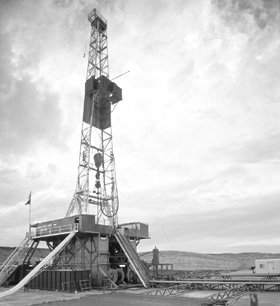Source: E. Dougherty, D. Dare, P. Hutchison, and E. Lombardino. "Optimizing SANTOS's Gas Production and Processing Operations in Central Australia Using the Decomposition Method," Interfaces 17, no. 1 (JanuaryFebruary 1987): 6593.

[Page 467]

The nonlinear programming model formulation for this problem is as follows: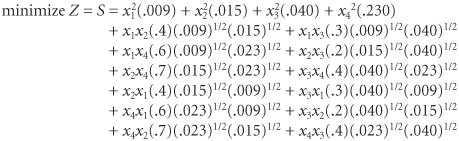subject to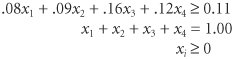The Excel spreadsheet for Jessica Todd's portfolio is shown in Exhibit 10.13. The decision variables representing the proportion of each stock in the portfolio ( x i ) are in cells E6:E9 . The formula for total return, =SUMPRODUCT ( B6:B9, E6:E9 ), is in cell B19. The objective is to minimize the total portfolio variance formula, which is shown on the formula bar at the top of the spreadsheet. This is a complicated formula and difficult to type in. Notice that it has been shortened slightly by doubling the covariance terms in the last part of the equation to reflect all the different investment pairs that are included in B14:E17 (i.e., 2 times AB, AC, AD, BC, BD, and CD will include BA, CA, DA, CB, DB, and DC). In other words, all the covariance terms to the right of the diagonal in the matrix B14:E17 are doubled to include the same values to the left of the diagonal.

##### Exhibit 10.13.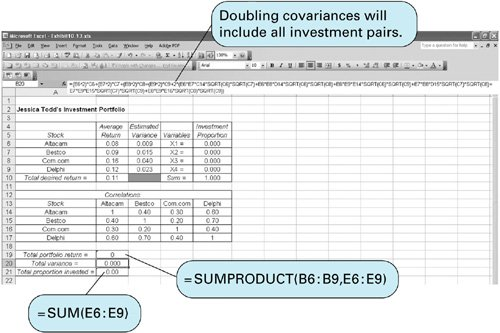The Solver Parameters window is shown in Exhibit 10.14. Notice that nonnegativity constraints for the variables are included E6:E90. The model solution is shown in Exhibit 10.15. It indicates that Jessica should invest 36.0% of her funds in Altacam ( x 1 ), 27.2% in Bestco ( x 2 ), 31.5% in Com.com ( x 3 ), and 5.3% in Delphi ( x 4 ). This will result in her desired minimum portfolio annual return of .11 and a variance of .0104. What does this variance mean? A variance of .0104 equals a standard deviation of .102. If returns are normally distributed (which is likely), then there is a .95 probability that the annual portfolio return will be between .089 {.11 (1.96)(.102)} and .310 {.11 + (1.96)(.102)}.

[Page 468]
##### Exhibit 10.14.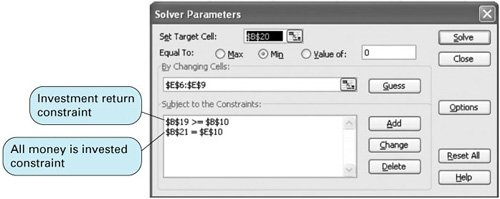##### Exhibit 10.15.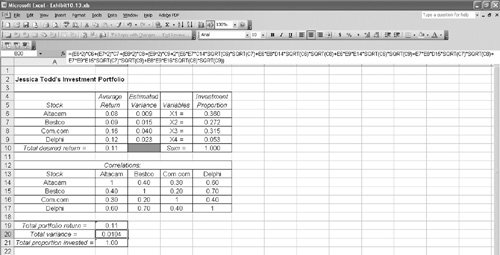Introduction to Management Science (10th Edition)
ISBN: 0136064361
EAN: 2147483647
Year: 2006
Pages: 358

Similar book on Amazon

flylib.com © 2008-2017.
If you may any questions please contact us: flylib@qtcs.net